﻿ HUCORE

## Why AI time-series?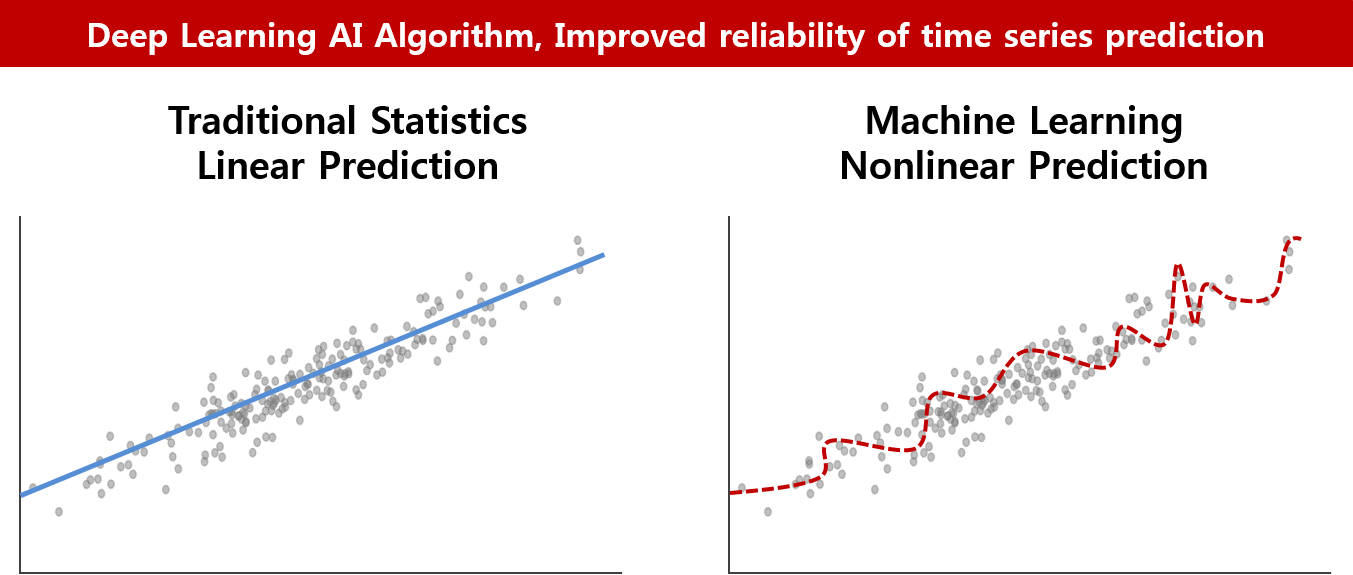### Improved reliability of time series prediction by utilizing sophisticated AI algorithms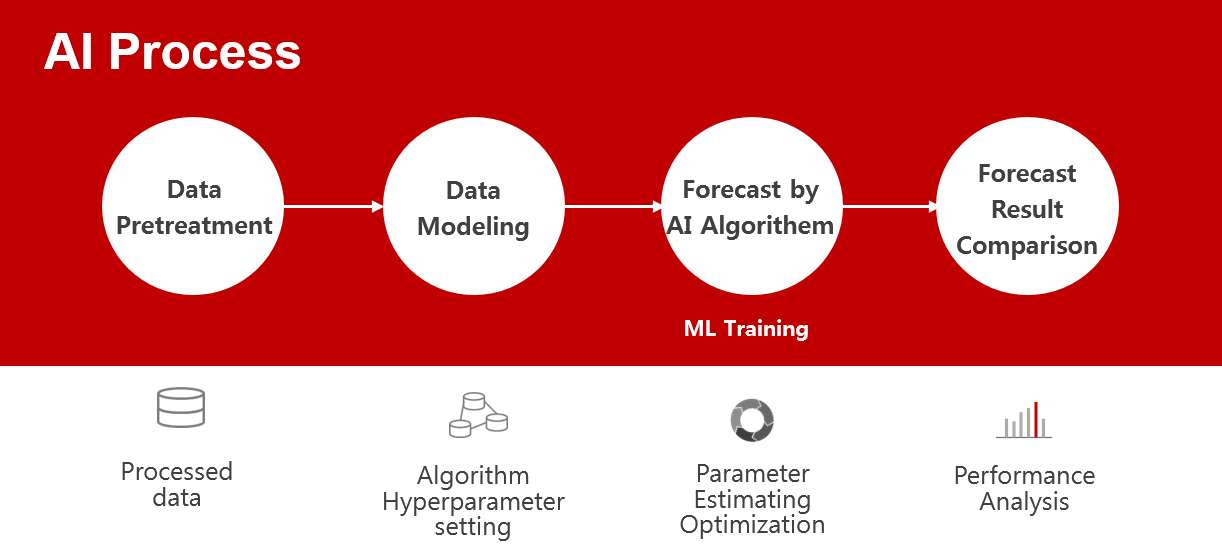## AI Modeling Process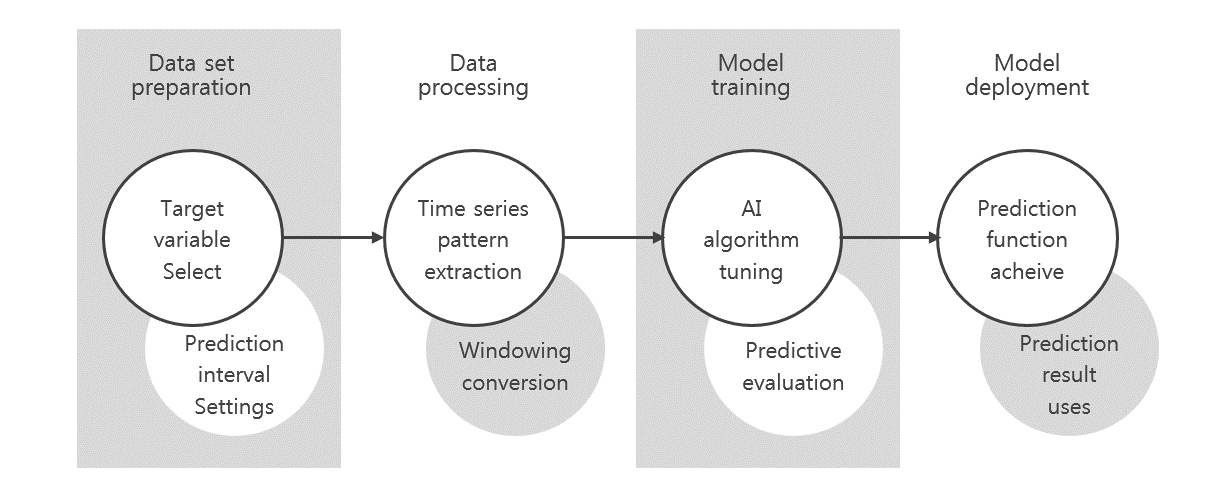## Time series algorithm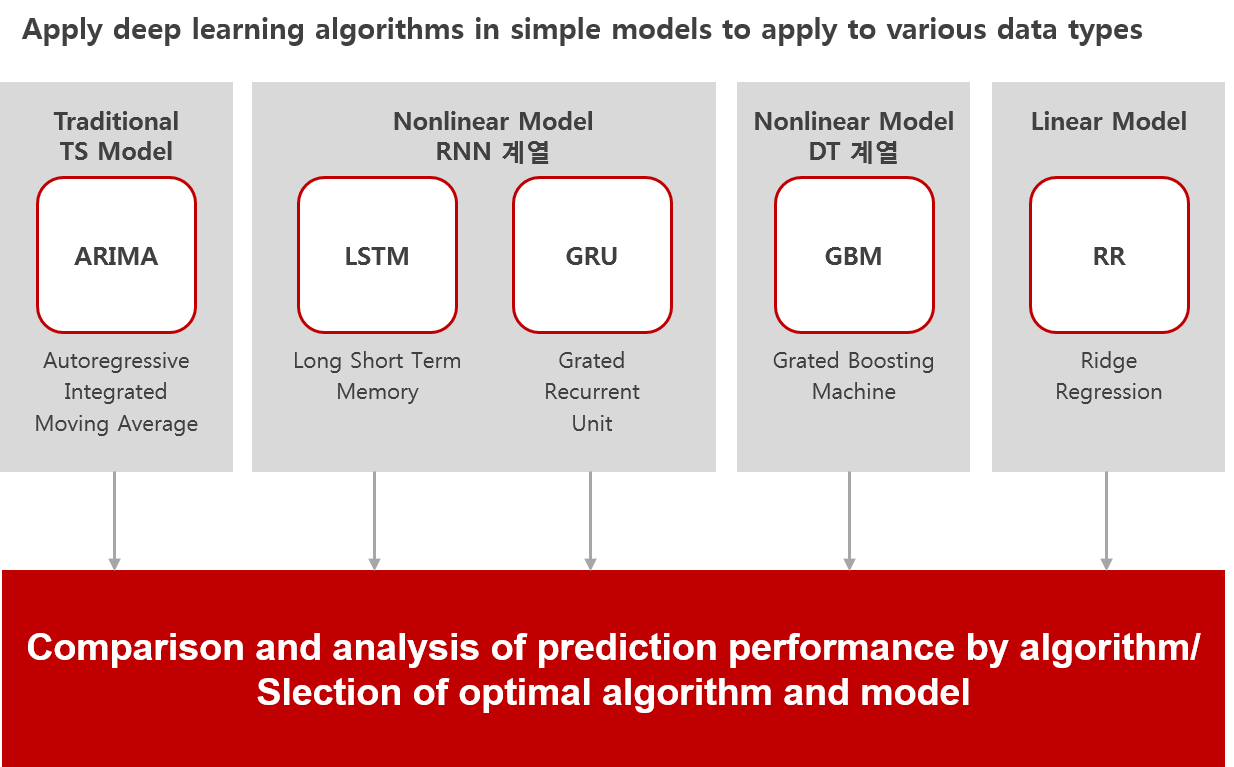## AI time-series Main Function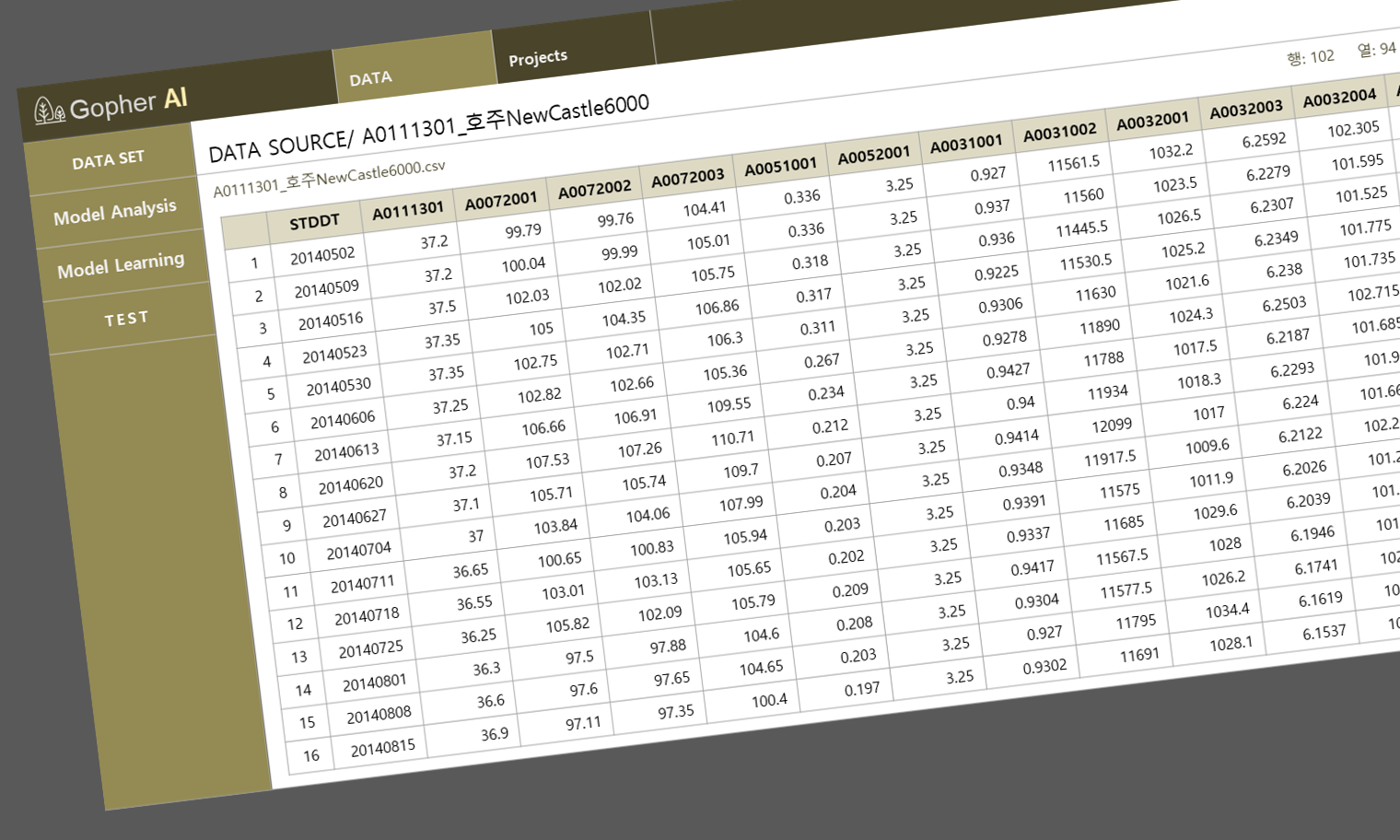• Data Interval processing, Resolving missing values
• Data pre-processing by data correlation analysis
• Create Target Field
• Modeling using various AI machine learning algorithms
• Champion Model derivation by model comparison analysis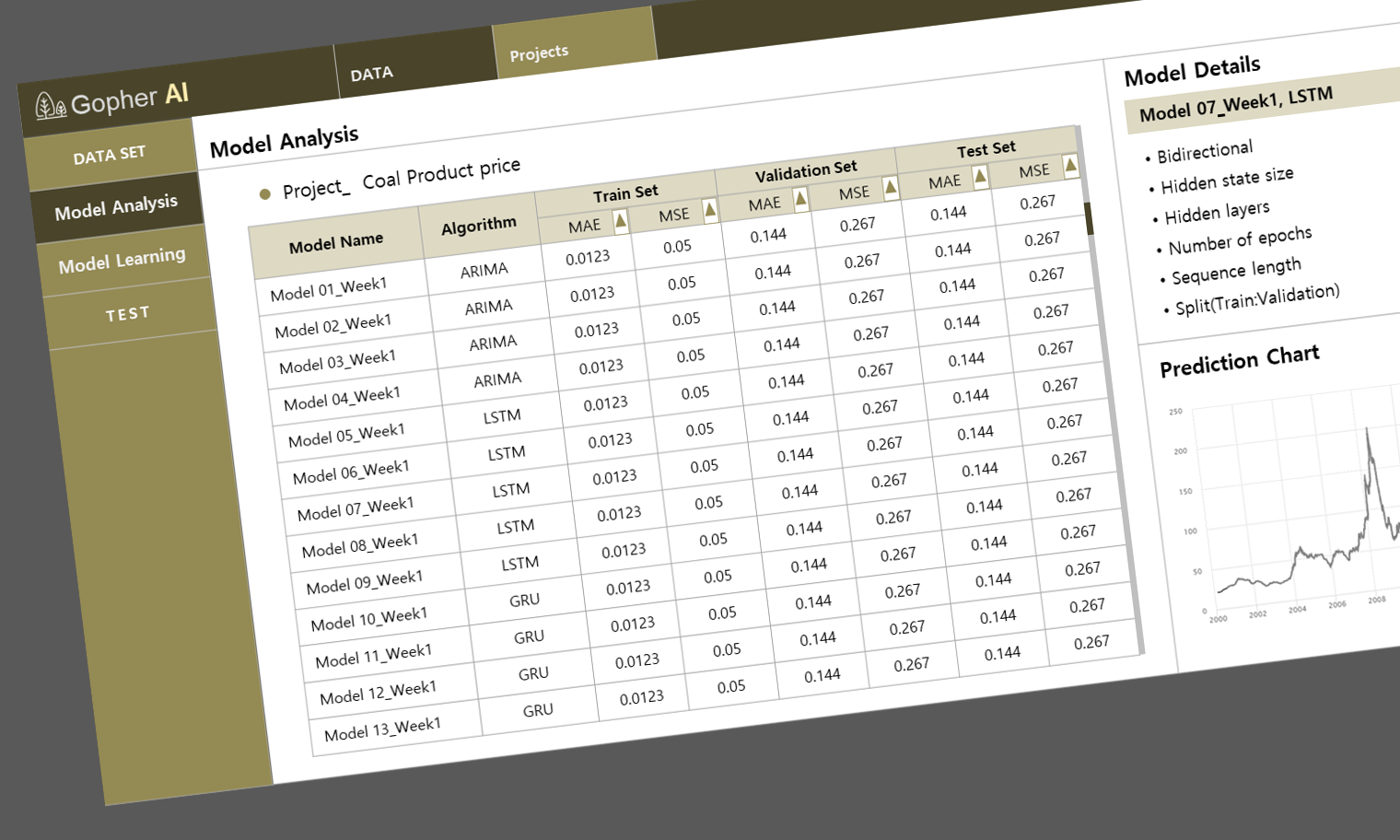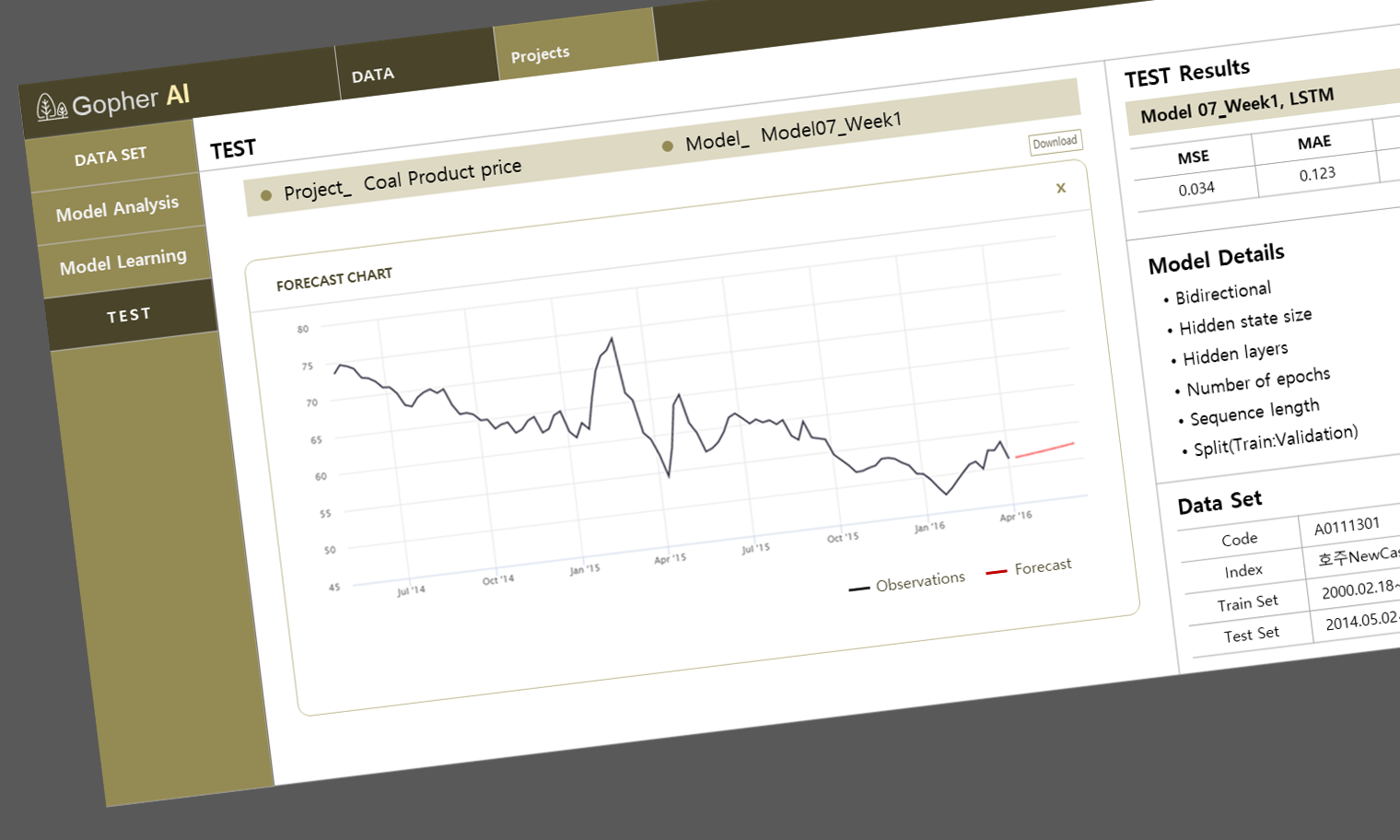• Applying a new data set to the optimal model
• Test performance verification of forecast results

## AI time-series Main Function• Data Interval processing, Resolving missing values
• Data pre-processing by data correlation analysis
• Create Target Field
• Modeling using various AI machine learning
algorithms
• Champion Model derivation by model comparison analysis• Applying a new data set to the optimal model
• Test performance verification of forecast results